# Newton’s Law of GravityPage 2

#### WATCH ALL SLIDES

The simulation will let me change the mass of the cannonball. Suppose we fire a 1 kg cannonball at 1 m/sec. Then we fire a 10 kg cannonball at 1 m/sec. What will happen?

A) The 10 kg cannonball will not travel as far as the 1 kg ball.

B) The 10 kg cannonball will travel the same distance.

C) The 10 kg cannonball will travel further than the 1 kg ball.

ConcepTest!

Slide 14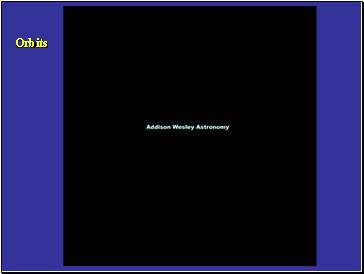Newton’s Law of Gravity

Orbits

Slide 15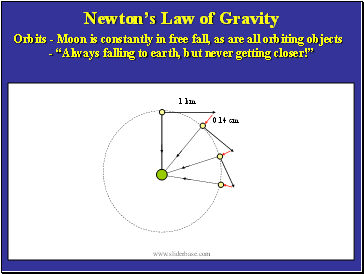Newton’s Law of Gravity

Orbits - Moon is constantly in free fall, as are all orbiting objects

- “Always falling to earth, but never getting closer!”

0.14 cm

1 km

Slide 16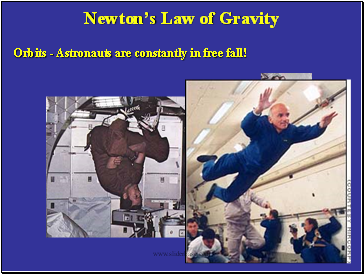Newton’s Law of Gravity

Orbits - Astronauts are constantly in free fall!

Slide 17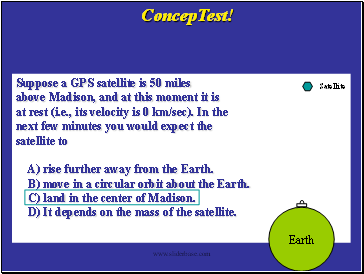Suppose a GPS satellite is 50 miles

above Madison, and at this moment it is

at rest (i.e., its velocity is 0 km/sec). In the

next few minutes you would expect the

satellite to

A) rise further away from the Earth.

B) move in a circular orbit about the Earth.

C) land in the center of Madison.

D) It depends on the mass of the satellite.

ConcepTest!

Earth

Satellite

Slide 18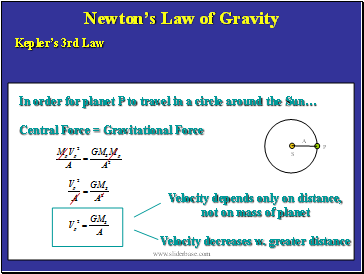Newton’s Law of Gravity

Kepler’s 3rd Law

A

S

P

In order for planet P to travel in a circle around the Sun…

Central Force = Gravitational Force

Velocity depends only on distance,

not on mass of planet

Velocity decreases w. greater distance

Slide 19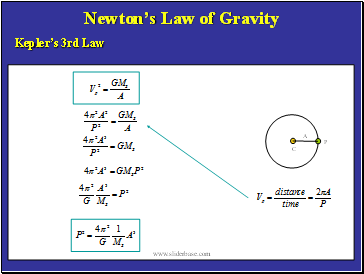Newton’s Law of Gravity

Kepler’s 3rd Law

A

C

P

Slide 20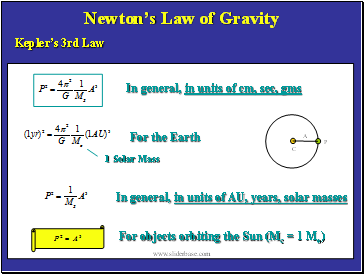Newton’s Law of Gravity

Kepler’s 3rd Law

In general, in units of cm, sec, gms

For the Earth

In general, in units of AU, years, solar masses

For objects orbiting the Sun (Mc = 1 Mo)

A

C

P

1 Solar Mass

Slide 21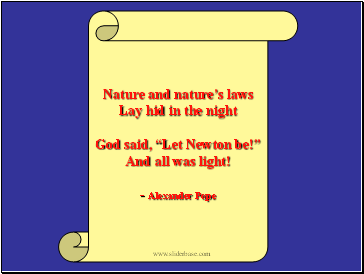Nature and nature’s laws

Lay hid in the night

God said, “Let Newton be!”

And all was light!

- Alexander Pope

Go to page:
1  2